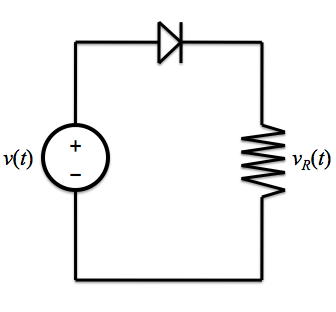# 1. Dark Characteristics

p-n junction diodes behave differently in the dark than they do when illuminated; this section explores their dark characteristics.

## Physical Characteristics

When not illuminated by a light source, such as the sun, the current running through a p-n junction diode can be calculated with the ideal diode equation:

i(v) = IS[exp(v / ηVT) - 1].

Although the ideal diode equation treats the reverse saturation current, IS, as a constant, it is actually a function of temperature. Experimental data shows that IS approximately doubles for every 10 K rise in temperature. Therefore, if IS is known at temperature T1, then IS at temperature T2 can be calculated with the following formula:

IS(T2) = 2(T2-T1)/10IS(T1).

Since IS is a function of temperature, it follows then that the resulting current, i, is also. According to Bobrow1, in order to keep a constant current i, the applied voltage must decrease by 3.5 mV for every 1 K increase.Temperature dependence of current in p-n junction diodes

## Circuit Applications

Since p-n junctions are nonlinear elements, analysis of circuits cannot be done using the ideal diode equation. Instead, the device is linearized by using the infinite step function approximation; that is, the diode is modeled as a switch that is ON when forward biased, or OFF when reverse biased. Consider the simple example of a sinusoidal, alternate current (AC) voltage source with a diode and resistor in series:Simple diode circuit

When the input voltage is positive, the diode is forward biased, and therefore it is ON. The diode then acts as a short circuit, and the voltage drop across the resistor is just equal to the voltage source. When the input voltage is negative, the diode is reverse biased, and therefore it is OFF. In this case, the diode acts as an open circuit and the voltage drop across the resistor is zero (see below). This simple device, which only allows positive voltages through the circuit, is a rectifier. A rectifier is so named because it "rectifies" or "corrects" alternating current (AC) into direct current (DC).The sinusoidal line is the input voltage; the red line (which is always nonnegative) is the rectified output voltage.
Image from Wikimedia Commons.

In order to solve circuits that can not be solved so simply, using this infinite step function model requires a guess-and-check approach: the diode is either assumed to be ON or OFF, the circuit is solved, and then the results are used to verify the prior assumptions. If the results support the assumptions, then the problem has been solved; if not, an alternate assumption must be made and the circuit solved again.

## References

1. "Chapter 6: Diodes." Fundamentals of Electrical Engineering. 2nd ed. New York, New York: Oxford UP, 1996. 363-74. Print.